Home > CC4 > Chapter 5 > Lesson 5.3.2 > Problem5-110

5-110.
1. Solve each equation. Homework Help ✎

1. (x + 2)(x + 3) = x2 – 10

2.x +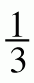x – 7 =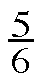x

3.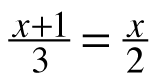4.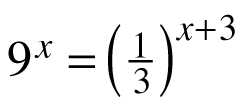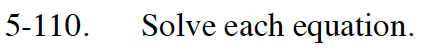Review the Math Notes box in Lesson 1.3.2 for the laws of exponents.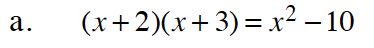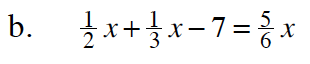no solution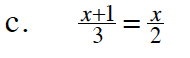Multiplying both sides by 6 will 'bust the fraction'.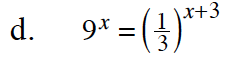32 can be substituted for the 9.

$3^{-1}\text{ can be substituted for }\frac{1}{3}$

$\left (3^{2} \right )^{x}=\left ( 3^{-1} \right )^{x+3}$